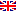Scheda programma d'esame
FISICA TEORICA 1
CdSFISICA
Codice213BB
CFU9
PeriodoPrimo semestre
LinguaItaliano

ModuliSettore/iTipoOreDocente/i
FISICA TEORICA 1FIS/02LEZIONI54Esporta in pdf
Learning outcomes
Knowledge

This course is an introduction to quantum field theories, which are generally used to describe fundamental interactions of particles and quantum many-body systems in condensed matter physics. In particular, the course deals with the construction of the field theory operators in Fock space, solutions of the Dirac equation,  computation of the transition amplitudes in perturbation theory. The course is supposed to provide the main tools to obtain quantitative information for high-energy processes, such as cross sections and decay rates of particles, at the leading order of perturbation theory using the technique of the Feynman diagrams, starting from given effective quantum field theories.

Assessment criteria of knowledge

In normal conditions, there is a final written examination and an oral examination. In the written exam (4 hours), where the student must solve a problem with several questions, he must demonstrate his/her ability in handling quantum field theories describing fundamental processes, and the methods to compute transition amplitudes. With the oral exam, the student must demonstrate the ability to approach a circumscribed research problem, and organise an effective exposition of the results.

Methods:

• Final oral exam
• Final written exam

Skills

Computation expertise in determining the values of observables in quantum field theories.

Assessment criteria of skills

Oral examination in which the student must demonstrate to be able to solve the proposed problems.

Prerequisites

Basic notions of quantum mechanics and relativistic kinematics.

Teaching methods

Delivery: face to face

Learning activities:

• attending lectures
• participation in discussions
• individual study
• group work

Teaching methods:

• Lectures
Programma (contenuti dell'insegnamento)

Transition amplitude and cross section for elastic scattering in Quantum Mechanics. Use of the Green function method applied to the Helmholtz equation and choice of the boundry conditions for the waave function of the outgoing scattered particles. Born approximation.

Second quantization, identical particles, Fock space, field operators in the interaction picture, time ordering, relativistic covariance for the fields, free lagrangian and interaction lagrangian, Noether theorem, continuous symmetries, groups U(1) and SU(2) and their representations.

Field operators, wave functions, solutions of the Dirac equation, scalar fields,  massless and massive vector fields, spinor fields, covariant bilinears, Weyl spinors, discrete symmetries.

Spinor electrodynamics, fields and lagrangian, gauge transformations and gauge invariance, minimal coupling, scalar electrodynamics.

S matrix, computation of the transition amplitudes in perturbation theory, derivation of the decay widths and of the cross sections in particles processes. Feynman propagator, Wick theorem, Feynman diagrams.

Syllabus

The main arguments are: second quantization, quantum field theory, invariant perturbation theory, Feynman diagrams for the calculation of matrix elements of scattering processes and decay rates within the theory of quantum electrodynamics, the standard model of electroweak interactions, and more generally for any quantum field theory defined by a local lagrangian. The course consists of about 60 classes, of which about a half are dedicated to the applications and problems.

Bibliography
•  M.E. Peskin and D.V. Schroeder,  An Inntroduction to Quantum Field Theory, Westview Press (Boulder, 1995).
• F. Mandl and G. Shaw, Quantum Field Theory, John Wiley & Sons (New York, 1984).

• C. Itzykson and J-B. Zuber, Quantum Field Theory, McGraw-Hill (Singapore, 1980).

• M. Maggiore, A Modern Introduction to Quantum Field Theory, Oxford University Press, (New York, 2005).

Ultimo aggiornamento 08/08/2022 16:13